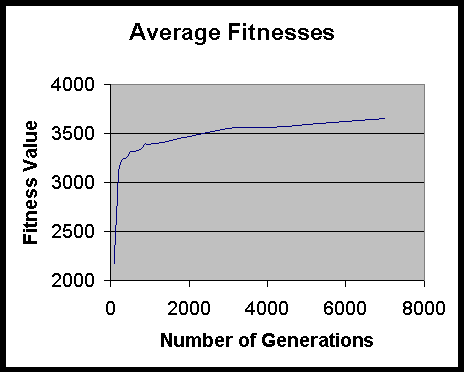# Introduction of genetic algorithm and 2.5 g and 3 g Networks

Jun 3 • Notes • 2329 Views • 2 Comments on Introduction of genetic algorithm and 2.5 g and 3 g Networks

Introduction of genetic algorithm and 2.5 g and 3 g Networks:-

Genetic Algorithm:-

An genetic algorithm (GA) is a search heuristic mechanism that mimics the process of natural evolution. This heuristic mechanism is routinely used to generate useful solutions to optimize and search problems. Genetic algorithms inherits from the larger class of evolutionary algorithm (EA), which generate solutions to optimization problems using techniques of natural evolution, such as inheritance, mutation, selection, and crossover.Fitness Function:-fitness function is a particular type of function which is objective that is used to summarize a single figure of merit that is, how close a given design solution is to achieving the aims. Moreover, the fitness function must not only correlate closely with the  goal put by the designers, it must be also be computed quickly. Speed of execution is very important, as in a typical genetic algorithm which must be iterated many times in order to produce a usable result for a non-trivial problem. There are two main classes of fitness functions exist: one where the fitness function does not change and, as in optimizing a fixed function or testing with a fixed set of test cases; and the other one where the fitness function is mutable.

Introduction to 2.5 g and 3 g Networks:-

The second generation cellular system are also called as 2G. 2G phase began in 1990s. 2G system are based on radio technologies which include frequency, code and time division multiple access. Examples of  2G systems are:- GSM (Europe), PDC (Japan), and IS-95(USA). circuit switched data links are used by 2g systems which have transmission speeds of 10-20 kbps up-link and down-link. The demand for higher data rates, the instant availability, the data volume-based charging and lack of radio spectrum allocated for 2G led to the introduction of 2.5G and 3G. Examples of 2.5G systems are:- GPRS and PDC-P. Examples of 3G systems are:- wideband CDMA  and CDMA2000. 3G systems provide both packet-switched and circuit-switched connectivity.

GATE Syllabus-

IES Syllabus-

### 2 Responses to Introduction of genetic algorithm and 2.5 g and 3 g Networks

1. Mrityunjay Kumar Singh says:

we are very familiar with the Genetic Algorithm, the fitness function. It will be very interesting, if we know the implementation of Genetic Algorithm.

2. swarn priya says: